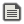# Does Petit computer have a ROUND()?StarryPenCreated:Does Petit computer have something like the ROUND() code from smilebasic?No but you can use modulo operator to do something like it. number % 1 gives you whatever is after the decimal point so you might do this:
`IF NUM%1<0.5 THEN NUM=FLOOR(NUM) ELSE NUM=FLOOR(NUM)+1`Replying to:snail_
No but you can use modulo operator to do something like it. number % 1 gives you whatever is after the decimal point so you might do this:
`IF NUM%1<0.5 THEN NUM=FLOOR(NUM) ELSE NUM=FLOOR(NUM)+1`
Oh alright thanks!